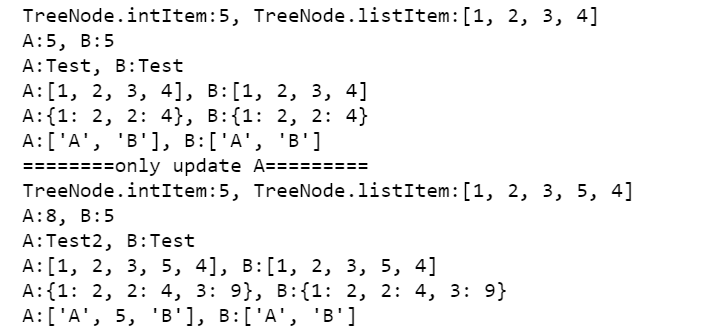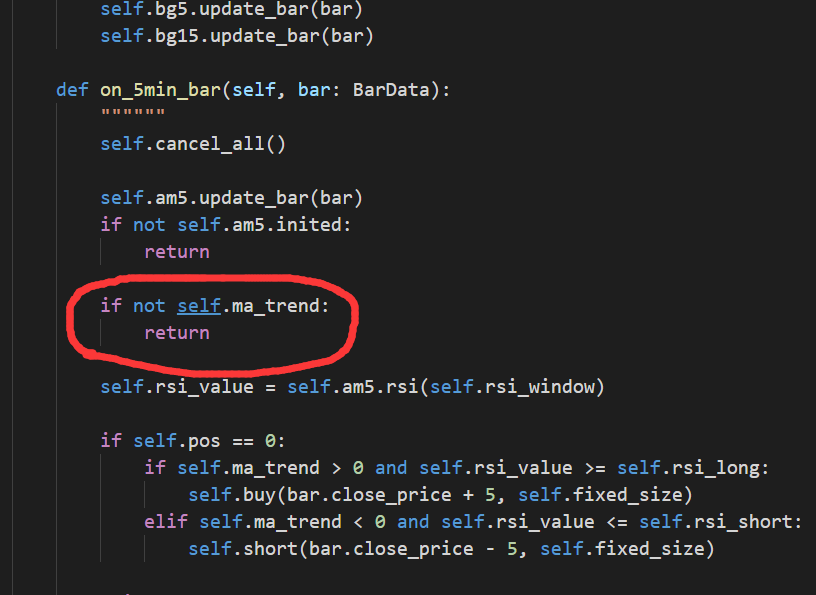VeighNa量化社区

``````class TreeNode:
intItem= 5
StringItem = 'Test'
listItem = [1,2,3,4]
Dict = {1:2,2:4}
def __init__(self, x):
self.Intval = x
self.listval= ['A','B']
A = TreeNode(1)
B = TreeNode(2)
print("TreeNode.intItem:%s, TreeNode.listItem:%s" %(TreeNode.intItem,TreeNode.listItem))
print("A:%s, B:%s" %(A.intItem, B.intItem))
print("A:%s, B:%s" %(A.StringItem, B.StringItem))
print("A:%s, B:%s" %(A.listItem, B.listItem))
print("A:%s, B:%s" %(A.Dict, B.Dict))
print("A:%s, B:%s" %(A.listval, B.listval))
A.intItem = 8
A.StringItem = 'Test2'
A.listItem.insert(-1,5)
A.Dict = 9
A.listval.insert(-1,5)
print("========only update A=========")
print("TreeNode.intItem:%s, TreeNode.listItem:%s" %(TreeNode.intItem,TreeNode.listItem))
print("A:%s, B:%s" %(A.intItem, B.intItem))
print("A:%s, B:%s" %(A.StringItem, B.StringItem))
print("A:%s, B:%s" %(A.listItem, B.listItem))
print("A:%s, B:%s" %(A.Dict, B.Dict))
print("A:%s, B:%s" %(A.listval, B.listval))``````